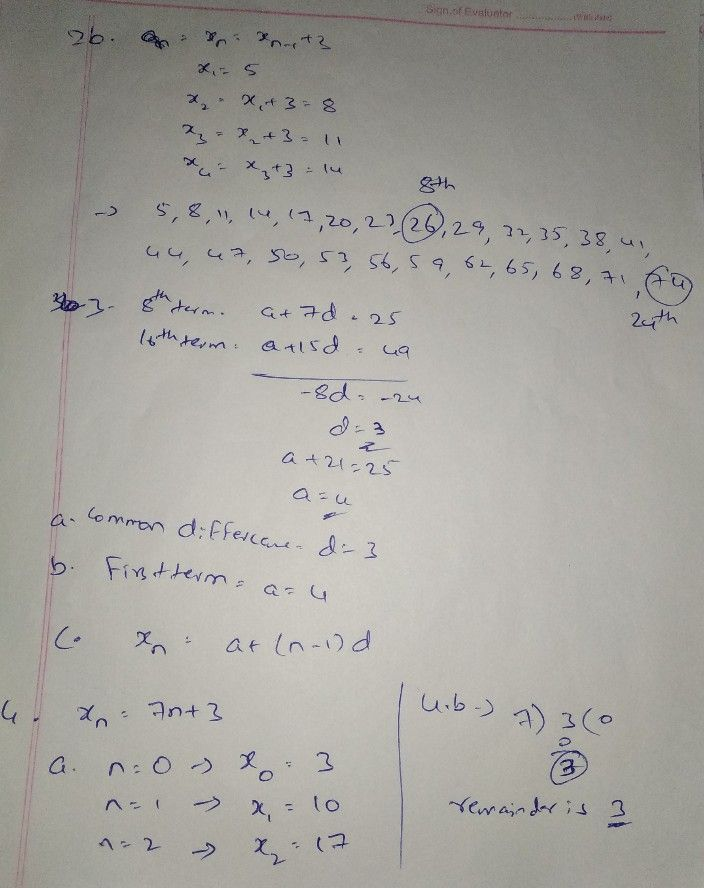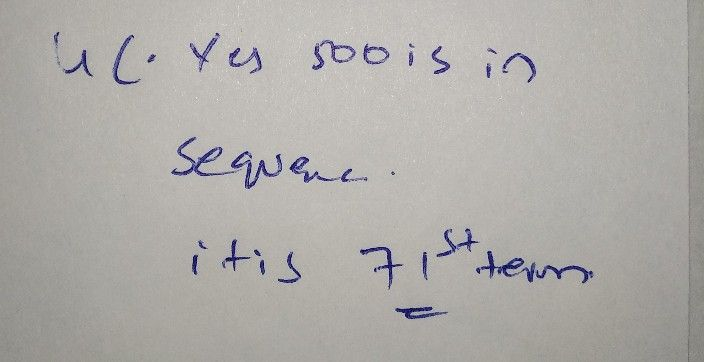Symbol
Problem$cncc$ $4$ 1. $a.$ Write an arithmetic sequence with common difference term of this sequence. b. What is the $15ih$ $te$ c. $ls$ $145$ the difference of any two terms of this sequence? Why? 2. a. Write the algebraic form of the arithmetic sequence $5,8,11..$ b. Write the 8th and $24th$ $te$ term of this sequence. 3. The 8th term of an arithmetic sequence $is25$ and 16th term is 49. a. What is the common difference? b. What is its first term? c. Write the algebraic form of this arithmetic sequence. 4. The algebraic form of an arithmetic sequence $1s$ $x_{n}=7n+3$ a. Write the first 3 terms. b. What is the remainder on dividing its terms by 7? c. Is $500,$ $a$ term of this sequence? Justify. 5. Consider arithmetic sequence 6, $11,$ $16,$ ... a. What is the common difference? b. Write the algebraic from of the sequence. terms? c. Find the sum of first $20term$ 6. The sum of 2nd and $30ih$ terms of an arithmetic $sc9$ $1cnCC$ $is50$ a. What is the sum of $1stand31st$ terms? b. What is the sum of $15nand$ 17th terms? c. What is the 16th term? $d$ What is the sum of first 31 terms?
10th-13th grade
Other
Search count: 106
SolutionQanda teacher - shivaramakplease ask the remaining questions separetly
if uncleared please do ask again